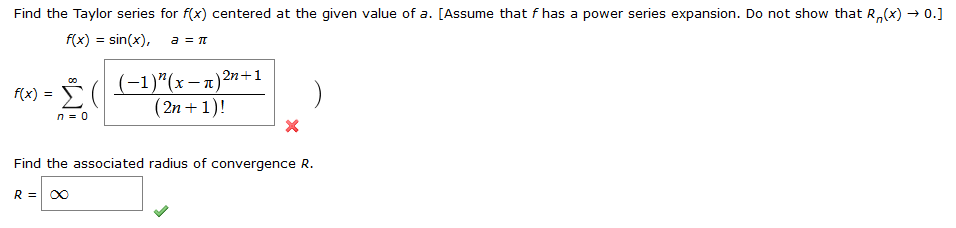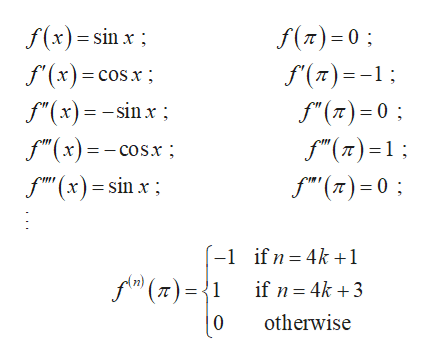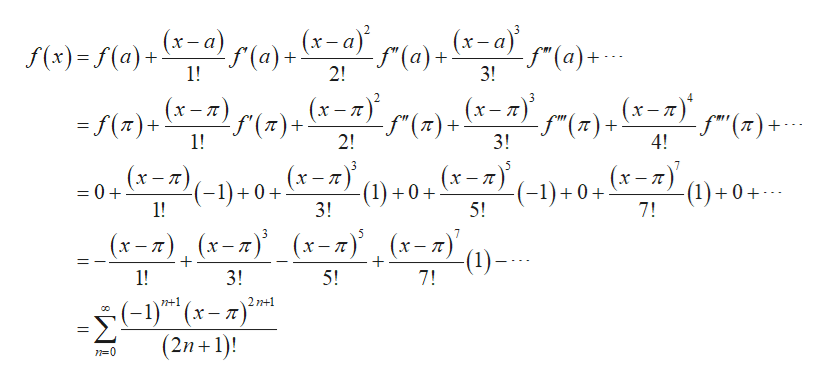# Find the Taylor series for f(x) centered at the given value of a. [Assume that f has a power series expansion. Do not show that R(x) 0.f(x) sin(x),a T(1)"(x-n)2n+1(2n + 1)!f(x) =n 0XFind the associated radius of convergence R.R =

Question
1 views

I followed all the steps for Maclauren series but got the wrong answer. Did I miss something?help_outlineImage TranscriptioncloseFind the Taylor series for f(x) centered at the given value of a. [Assume that f has a power series expansion. Do not show that R(x) 0. f(x) sin(x), a T (1)"(x-n)2n+1 (2n + 1)! f(x) = n 0 X Find the associated radius of convergence R. R = fullscreen
check_circle

star
star
star
star
star
1 Rating
Step 1

Compute the derivative of the function and its value at a =π.help_outlineImage Transcriptionclosef(x) sinx f() 0 f'(x)=coSx; f() f(x)sinx f"(x)=-cos.x f(x)sin x f(n)0 f(T)0 -1 ifn = 4k +1 f(T)1 if n 4k3 otherwise 0 fullscreen
Step 2

Apply the values in Taylor series expa...help_outlineImage Transcriptionclose(x-a) f(a) 1! (a)+ (x-a) -f"(a) 3! f(x)=f(a)+(-a) 2! (x-)() (x-n (x-nfn)+ (x-T) =f()+ 1! f"(7)+ 2! f"(77)+ 3! f"'"(7)+ 4! (x-z (x-7) 7 (x-7 |(x-T -(-1)+0+ (1)+0 7! -1)+0+ 1! (1)+0+ 5! 0+ 3! 5 (*-т), (х-т) (х-т)' (х-т) 1! 3! 5! 7! -(1)- 1) -Σ (2n+1) n-0 fullscreen

### Want to see the full answer?

See Solution

#### Want to see this answer and more?

Solutions are written by subject experts who are available 24/7. Questions are typically answered within 1 hour.*

See Solution
*Response times may vary by subject and question.
Tagged in

### Math